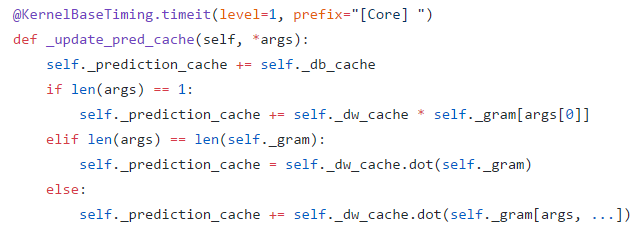（这里是本章会用到的 Jupyter Notebook 地址）

（考试周写专栏真有种忙里偷闲的感觉 _(:з」∠)_）

## 什么是核方法？• 将算法表述成样本点内积的组合（这经常能通过算法的对偶形式实现）
• 设法找到核函数，它能返回样本点作用后的内积
•替换、完成低维到高维的映射（同时也完成了从线性算法到非线性算法的转换）

Mercer 定理为寻找核函数带来了极大的便利。可以证明如下两族函数都是核函数：

• 多项式核## 怎么应用核方法？（也有令的）（有没有发现核形式和对偶形式很像？( σ'ω')σ）

## 如何训练核模型？

【注意：为简洁，从此往后的推导和实现均以核感知机为例，核 SVM 的相关讨论会放在下一章介绍 SMO 算法时进行】# 假设 k_mat 存储着原样本之间的核矩阵
# 1、计算损失
err = -y * (k_mat.dot(alpha) + b)
# 2、找出使得损失不小于 0 的样本
# 3、进行相应梯度下降，lr 是学习速率
alpha += np.sum(delta[..., None] * k_mat[mask], axis=0)
b += np.sum(delta)@staticmethod
def _poly(x, y, p):
return (x.dot(y.T) + 1) ** p

@staticmethod
def _rbf(x, y, gamma):
return np.exp(-gamma * np.sum((x[..., None, :] - y) ** 2, axis=2))

## 核模型的实现

• 需要定义核函数并计算出核矩阵
• 计算预测值时不是，而是，其中
• 在训练时，为原样本之间的核矩阵
• 在测试时，为原样本和新样本的核矩阵

# 训练代码
def fit(...):
...
self._alpha = np.zeros(len(x))
self._b = 0.
self._x = x
# self._kernel 即为核函数，能够计算两组样本的核矩阵
k_mat = self._kernel(x, x)
for _ in range(epoch):
err = -y * (self._alpha.dot(k_mat) + self._b)
if np.max(err) < 0:
continue
self._alpha += np.sum(delta[..., None] * k_mat[mask], axis=0)
self._b += np.sum(delta)

# 预测代码
def predict(self, x, raw=False):
x = np.atleast_2d(x).astype(np.float32)
# 计算原样本与新样本的核矩阵并根据它来计算预测值
k_mat = self._kernel(self._x, x)
y_pred = self._alpha.dot(k_mat) + self._b
if raw:
return y_pred
return np.sign(y_pred).astype(np.float32)

## 相关数学理论

1）Cover 定理2）Mercer 定理是对称函数（亦即）的话，那么它具有 Hilbert 空间中内积形式的充要条件有以下两个：

• 对任何平方可积的函数、满足• 对含任意 N 个样本的数据集，核矩阵：是半正定矩阵

【注意：通常我们会称满足这两个充要条件之一的函数为 Mercer 核函数而把核函数定义得更宽泛。不过如果不打算在理论上深入太多的话，将 Mercer 核函数简称为核函数是可以的。此外，虽说 Mercer 核函数确实具有 Hilbert 空间中的内积形式、但此时的 Hilbert 空间并不一定具有“维度”这么好的概念（或说、可以认为此时 Hilbert 空间的维度为无穷大；比如说 RBF 核，它映射后的空间就是无穷维的）】

3）表示定理为核函数对应的映射后的空间（RKHS），表示的范数，那么对于任意单调递增的函数和任意非负损失函数、优化问题（猛戳我进入下一章！( σ'ω')σ ）